• 提供一种适用于初中级学者的时间序列预测模型 并且十分的有效好用 数据介绍 该数据集是一个污染数据集，我们需要用该多维时间序列去预测pollution这个维度，采用80%作为训练集，20%作为测试集。 模型实现 模型使用...
朋友们好，时隔很久我又开始写时间序列相关的博客啦。
新年新气象,过去的时间序列预测博客采用版本为Keras  2.2  tensorflow-gpu 1.13.1版本实现。
本次博客的主题是：
提供一种适用于新手的LSTM时间序列预测模型
十分的有效好用
同时采用Keras 2 + TensorFlow 2  实现，提供预测和验证全流程。
版本:
cuda  10.1
cudnn 8.0.5
keras 2.4.3
tensorflow-gpu 2.3.0
Keras 2.4版本仅支持TensorFlow 作为后端，参见 Keras 2.4发布，真正成为TensorFlow的Keras ，import 相比之前版本也有一些改动。
数据介绍
该数据集是一个污染数据集，我们需要用该多维时间序列去预测pollution这个维度，采用80%作为训练集，20%作为测试集。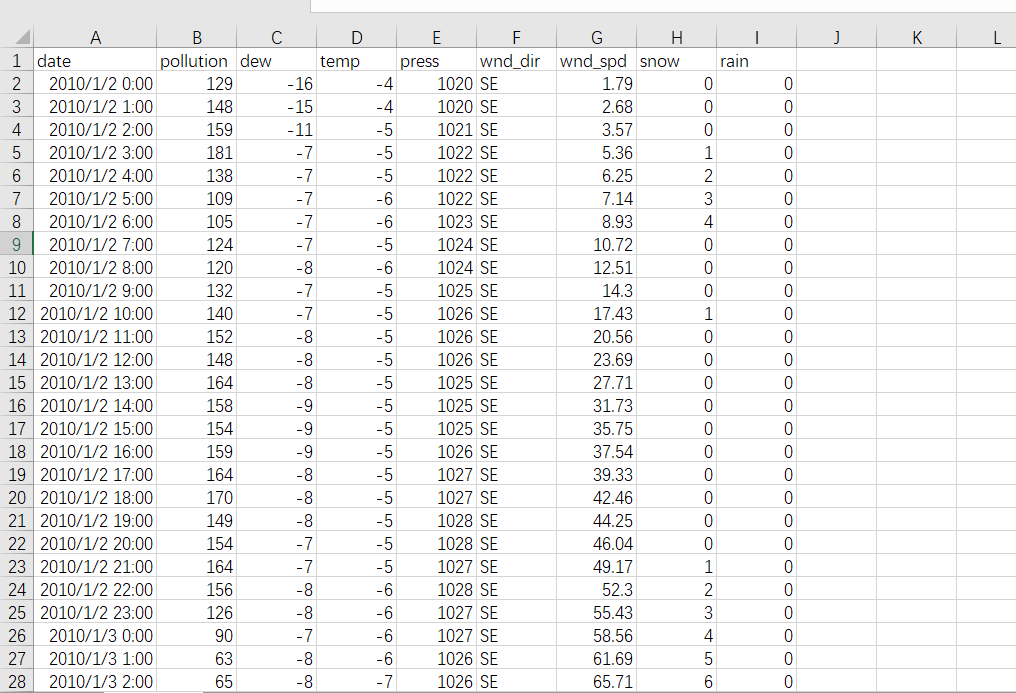开始
深度学习的第一步
import tensorflow as tf

相对于旧版本keras 这里有一些改动
from  tensorflow.keras import Sequential
from  tensorflow.keras.layers import LSTM,Dense,Activation,Dropout
from  tensorflow.keras.callbacks import History,Callback,EarlyStopping
import  numpy as np

模型实现
模型使用最简单的序贯模型，
使用双层LSTM加一个全连接层实现预测
具体结构如下
然后还添加了一个early stopping 机制

def lstm_model(train_x,train_y,config):

model = Sequential()
return_sequences=True))

config.lstm_layers,
return_sequences=False))

train_y.shape))

model.summary()

cbs = [History(), EarlyStopping(monitor='val_loss',
patience=config.patience,
min_delta=config.min_delta,
verbose=0)]
model.compile(loss=config.loss_metric,optimizer=config.optimizer)
model.fit(train_x,
train_y,
batch_size=config.lstm_batch_size,
epochs=config.epochs,
validation_split=config.validation_split,
callbacks=cbs,
verbose=True)
return model

同时具体模型的输入输出是根据train_x和 train_y的shape来设置的。所以这是一个自适应的模型 。
只要确保train_x的维度为3，train_y的维度为2，就能流畅运行。
具体参数
#使用类实现一个配置文件
class Config:
def __init__(self):
self.path = './Model/'
self.dimname = 'pollution'

#使用前n_predictions 步去预测下一步
self.n_predictions = 30

#指定EarlyStopping  如果训练时单次val_loss值不能至少减少min_delta时，最多允许再训练patience次
#能够容忍多少个epoch内都没有improvement
self.patience = 10
self.min_delta = 0.00001

#指定LSTM两层的神经元个数
self.lstm_layers = [80,80]
self.dropout = 0.2

self.lstm_batch_size = 64
self.loss_metric = 'mse'
self.validation_split = 0.2
self.verbose = 1
self.epochs = 200

## 是一个数组 如[64,64]
def change_lstm_layers(self,layers):
self.lstm_layers = layers


模型结构如图由于采用了Early Stopping机制 训练28次就结束了
Epoch 28/200
438/438 [==============================] - 10s 23ms/step - loss: 8.4697e-04 - val_loss: 4.9450e-04
结果
让我们来看看结果吧
RMSE为 24.096020043963737
MAE为 13.384563587562422
MAPE为 25.183164455025054可以看到我们这个方法虽然简单，但是预测效果是很好的~
注
本代码已经上传到了我的github
同时还附录了本教程的旧版本(梯度搜索部分可能有点小bug 如果使用需要仔细校对一下)
如果本文点赞过1000或者github 本项目 star 过100
我就开源 登堂入室LSTM：使用LSTM进行简单的时间序列异常检测
的新版本 更优实现。
参考
tensorflow2_tutorials_chinese
Anomaly Detection in Time Series Data Using LSTMs and Automatic Thresholding


展开全文lstm 深度学习 keras
• SVM_CAR首先利用SVM以留一法的MSE最小化原则进行时间序列非线性定阶；然后用SVM对害虫发生的影响因子进行非线性筛选，并同时通过强制汰选给出各保留因子对预测结果的相对重要性；最后建立基于保留对预测结果影响较大...支持向量机 非线性
• 时间序列预测， 传统模型：比如ARIMA模型，一次只能对一组时间序列数据进行预测，比如预测某个品牌下某家店未来销售额。 现实情况中需要预测某个品牌下每家店未来的销售额。也就是说，如果这个品牌在某地区一共...
另一篇博文地址：时间序列arima预测：https://blog.csdn.net/u014281392/article/details/77585419
做时间序列预测，
传统模型：比如ARIMA模型，一次只能对一组时间序列数据进行预测，比如预测某个品牌下某家店未来销售额。
现实情况中需要预测某个品牌下每家店未来的销售额。也就是说，如果这个品牌在某地区一共有100家店，我们就需要给出这100家店分别对应的销售额预测值。
此时传统模型便不再适合。
时间序列预测模型建模思路

初始数据集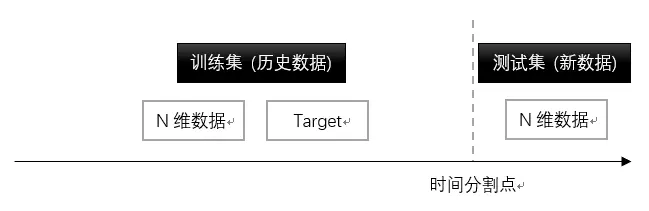一开始拿到的数据可能是分好训练集、测试集的，也可能是没分好的。这里我按照竞赛的情况假设一开始的数据集分有训练集和测试集。

对于时间序列数据来说，训练集即为历史数据，测试集即为新数据。历史数据对应的时间均在时间分割点之前（如2018年以前某品牌每家店每天的销售数据），新数据对应的时间均在分割点之后（如2018年以后某品牌每家店每天的销售数据）。
历史数据和新数据均包含N维信息（如某品牌每家店的地理位置、销售的商品信息等），但前者比后者多一列数据：Target，即要预测的对象，如销售额。
基于给出的数据，我们的预测任务是：根据已有数据，预测测试集的Target（如，根据某品牌每家店2018年以前的历史销售情况，预测每家店2018年1月份头15天的销售额）

数据处理
在构建预测特征上，截面数据和时间序列数据遵循的逻辑截然不同。下面两张图分别是二者的数据处理逻辑示意图。

首先来看针对截面数据的数据处理思路。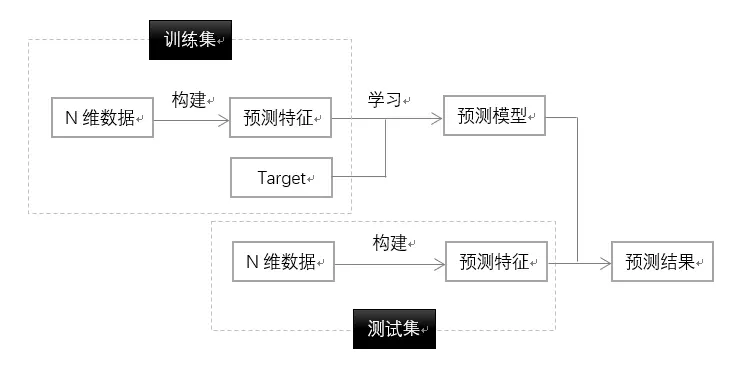对于截面数据来说，训练集数据和测试集数据在时间维度上没有区别，二者唯一的区别是前者包含要预测的目标变量，而后者没有该目标变量。
一般来说，在做完数据清洗之后，我们用“N维数据”来分别给训练集、测试集构建M维预测特征（维度相同），然后用机器学习算法在训练集的预测特征和Target上训练模型，最后通过训练出的模型和测试集的预测特征来计算预测结果（测试集的Target）。
此外，为了给模型调优，我们一般还需要从训练集里面随机分割一部分出来做验证集。
而时间序列的处理思路则有所不同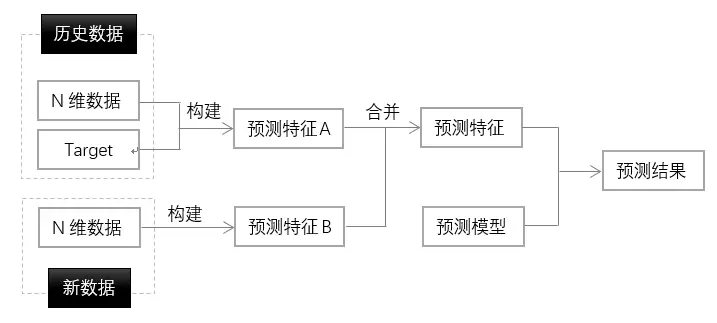时间序列预测的核心思想是：用过去时间里的数据预测未来时间里的Target。
所以，在构建模型的时候，所有过去时间里的数据（训练集里的N维数据和Target，如2018年以前每家店的地理信息、所卖商品信息、日销售额等）都应该拿来构建预测特征。
而新数据本身的N维数据（如2018年1月头15天每家店的地理信息、所卖商品信息等）也应该拿来构建预测特征。
前者是历史特征（对应图上的预测特征A），后者是未来特征（对应图上的预测特征B）。二者合起来构成总预测特征集合。
最后，用预测模型和这个总的预测特征集合来预测未来Target（如未来销售额）。
看到这里，一个问题就产生了：既然所有的数据都拿来构建预测特征了，那预测模型从哪里来？没有Target数据，模型该怎么构建？
你可能会说，那就去找Target呗。对，没有错。但这里需要注意，我们要找的不是未来时间下的Target（毕竟未来的事还没发生，根本无从找起），而是从过去时间里构造“未来的”Target，从而完成模型的构建。这是在处理时间序列上，逻辑最绕的地方。
3. 模型构建
用机器学习算法构造时间序列预测模型，关键的思路在于，通过时间滑窗，人为地构造“未来”Target，来给算法进行学习。
有点绕，请看下面的示意图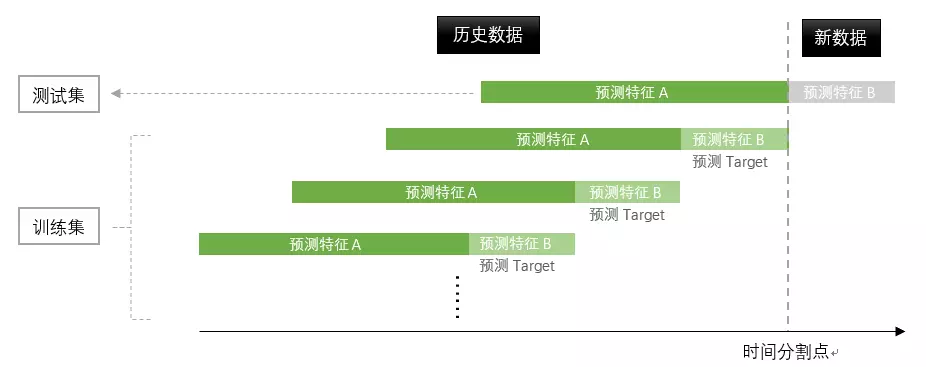和之前一样，从时间的角度上来看，我们有历史数据，和新数据。但这里，我们不能简单地把历史数据作为训练集、把新数据作为测试集。
怎么做呢。
首先，在历史数据上，我们通过截取不同时间窗口的数据来构造一组或几组数据。比如，我的历史数据是2017年 1月到12月每家店每天的销售数据，那么我可以截取3组数据（见上图的深绿、浅绿部分）：2017年1月到10月的数据、2017年2月到11月的数据、2017年3月到12月的数据。
然后，人为地给每组数据划分历史窗口（对应上图的深绿色部分）和未来窗口（对应上图的浅绿色部分）。比如，对于2017年1月到10月的数据，我把1月到9月作为历史窗口、10月作为未来窗口，以此类推。
接着，分别给每组数据构建预测特征，包括历史特征（预测特征A）和未来特征（预测特征B）。而此时，每组数据还有预测Target。
这个时候，我们把得到的所有预测特征（我的例子里是三组预测特征）都合并起来作为训练集特征、把所有预测Target（我的例子里是三组预测Target）合并起来作为训练集Target，之后就可以构建机器学习模型了。
有了训练集和训练模型，还差测试集。测试集的构建遵循之前的数据处理逻辑，拿历史数据构建历史特征，拿新数据构建未来特征，然后把这些特征加入到从训练集上训练出的预测模型中去，即可得到任务需要的最终预测值。
这里需要注意，划多少个时间窗口因数据而异。此外，数据的历史窗口（图上深绿部分）和未来窗口（图上浅绿部分）可以是定长也可以是变长，看具体情况。
以上就是我最近总结出的用机器学习算法构建时间序列预测模型的建模思路。
时间序列预测竞赛代码：
https://github.com/wepe/O2O-Coupon-Usage-Forecast
转载来源：https://blog.csdn.net/weixin_42587745/article/details/82965531


展开全文• 原文链接 from math import sqrt from numpy import concatenate from matplotlib import pyplot from pandas import read_csv from pandas import DataFrame from pandas import concat from sklearn....
原文链接

from math import sqrt
from numpy import concatenate
from matplotlib import pyplot
from pandas import DataFrame
from pandas import concat
from sklearn.preprocessing import MinMaxScaler
from sklearn.preprocessing import LabelEncoder
from sklearn.metrics import mean_squared_error
from keras.models import Sequential
from keras.layers import Dense
from keras.layers import LSTM
# convert series to supervised learning

from datetime import datetime
def parse(x):
return datetime.strptime(x, '%Y %m %d %H')
dataset = read_csv('lg.csv',  parse_dates = [['year', 'month', 'day', 'hour']], index_col=0, date_parser=parse)
dataset.drop('No', axis=1, inplace=True)
# manually specify column names
dataset.columns = ['pollution', 'dew', 'temp', 'press', 'wnd_dir', 'wnd_spd', 'snow', 'rain']
dataset.index.name = 'date'
# mark all NA values with 0
dataset['pollution'].fillna(0, inplace=True)
# drop the first 24 hours
dataset = dataset[24:]
# summarize first 5 rows
# save to file
dataset.to_csv('pollution.csv')

def series_to_supervised(data, n_in=1, n_out=1, dropnan=True):
n_vars = 1 if type(data) is list else data.shape
df = DataFrame(data)
cols, names = list(), list()
# input sequence (t-n, ... t-1)
for i in range(n_in, 0, -1):
cols.append(df.shift(i))
names += [('var%d(t-%d)' % (j+1, i)) for j in range(n_vars)]
# forecast sequence (t, t+1, ... t+n)
for i in range(0, n_out):
cols.append(df.shift(-i))
if i == 0:
names += [('var%d(t)' % (j+1)) for j in range(n_vars)]
else:
names += [('var%d(t+%d)' % (j+1, i)) for j in range(n_vars)]
# put it all together
agg = concat(cols, axis=1)
agg.columns = names
# drop rows with NaN values
if dropnan:
agg.dropna(inplace=True)
return agg
values = dataset.values
# integer encode direction
encoder = LabelEncoder()
values[:,4] = encoder.fit_transform(values[:,4])
# ensure all data is float
values = values.astype('float32')
# normalize features
scaler = MinMaxScaler(feature_range=(0, 1))
scaled = scaler.fit_transform(values)
# frame as supervised learning
reframed = series_to_supervised(scaled, 1, 1)
# drop columns we don't want to predict
reframed.drop(reframed.columns[[9,10,11,12,13,14,15]], axis=1, inplace=True)
# split into train and test sets
values = reframed.values
n_train_hours = 365 * 24
train = values[:n_train_hours, :]
test = values[n_train_hours:, :]
# split into input and outputs
train_X, train_y = train[:, :-1], train[:, -1]
test_X, test_y = test[:, :-1], test[:, -1]
# reshape input to be 3D [samples, timesteps, features]
train_X = train_X.reshape((train_X.shape, 1, train_X.shape))
test_X = test_X.reshape((test_X.shape, 1, test_X.shape))
print(train_X.shape, train_y.shape, test_X.shape, test_y.shape)
# design network
model = Sequential()
# fit network
history = model.fit(train_X, train_y, epochs=50, batch_size=72, validation_data=(test_X, test_y), verbose=2, shuffle=False)
# plot history
pyplot.plot(history.history['loss'], label='train')
pyplot.plot(history.history['val_loss'], label='test')
pyplot.legend()
pyplot.show()
# make a prediction
yhat = model.predict(test_X)
test_X = test_X.reshape((test_X.shape, test_X.shape))
# invert scaling for forecast
inv_yhat = concatenate((yhat, test_X[:, 1:]), axis=1)
inv_yhat = scaler.inverse_transform(inv_yhat)
inv_yhat = inv_yhat[:,0]
# invert scaling for actual
inv_y = scaler.inverse_transform(test_X)
inv_y = inv_y[:,0]
# calculate RMSE
rmse = sqrt(mean_squared_error(inv_y, inv_yhat))
print('Test RMSE: %.3f' % rmse)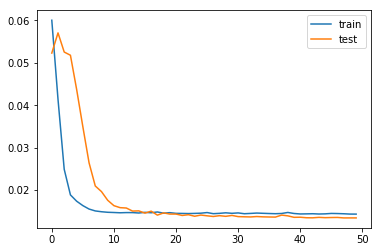代码下载
展开全文• <br />多维数据挖掘结构 select flattened [Store Forecasting].[Store], PredictTimeSeries([Store Forecasting].[Time],12) as t --此处只能使用Store Forecasting].[Time]作为输入列 from ...多维数据挖掘结构
select flattened [Store Forecasting].[Store],PredictTimeSeries([Store Forecasting].[Time],12) as t                    --此处只能使用Store Forecasting].[Time]作为输入列from [Store Forecasting]

参考文章：http://msdn.microsoft.com/zh-cn/library/ms132167.aspx
展开全文数据挖掘
• 现在我总结一下常用的LSTM时间序列预测： 1.单维单步（使用前两步预测后一步） 可以看到trainX的shape为 (5,2) trainY为(5,1) 在进行训练的过程中要将trainX reshape为 (5,2,1)(LSTM的输入为 [samples, ...LSTM 时间序列 时间序列预测 多维时间序列
• 针对非线性时间序列, 提出一种基于多维泰勒网的时间序列预测方法. 其特点在于利用非线性时间序列的观测数据, 通过多维泰勒网得到?? 元一阶多项式差分方程组, 在无需待预测系统的任何先验知识和机理的情况下获得动力...
• 基于SVR的多维时间序列分析及其在农业科学中的应用，袁哲明，张永生，【目的】建立一种基于结构风险最小、既反映样本集动态特征又体现环境因子影响的高精度非线性多维时间序列预测方法。【方法】耦合
• 基于多维时间序列的数控加工中心运行状态预测方法研究，张彦如，邓广周，对现代企业数控加工中心运行状态预测是进行故障预警、事前维护、保障设备高效运行的关键。目前针对设备状态预测的研究很多，但是
• 1.单维单步（使用前两步预测后一步） 可以看到trainX的shape为 (5,2) trainY为(5,1) 在进行训练的过程中要将trainX reshape为 (5,2,1)(LSTM的输入为 [samples, timesteps, features] 这里的timesteps为步数，...
• 基于小波和多维泰勒网动力学模型的金融时间序列预测研究论文
• 计划用两篇文章介绍如何开发 1D CNN 进行多步时间序列预测。主要内容如下： 如何为单变量数据开发多步时间序列预测的CNN模型； 如何为多变量数据开发多通道多步时间序列预测的CNN模型； 如何为多变量数据开发多头...
• 数据集 首先介绍一下我们的数据集，可以在我的github下载 该数据集是一个污染数据集，我们需要用该多维时间序列预测pollution这个维度 构建训练数据 首先我们删去数据中date,wnd_dir维（注:为了演示方便故不使用...深度学习 LSTM 注意力机制 Keras
• 论文研究-基于小波和多维泰勒网动力学模型的金融时间序列预测.pdf, 提出建立多维泰勒网动力学模型及参数辨识方法, 和基于小波多维泰勒网模型的金融时间序列预测方法. ...
• 【论文分享】–多维时间序列异常检测 时序异常检测算法概览 AutoEncoder 是一种典型的无监督方法，可以将其扩展为 variational AutoEncoder，或者引入情景信息，从而扩展为 Conditional Variational AutoEncoder...时序 异常检测
• 单变量时间序列预测 数据类型：单列 ​ import numpy import matplotlib.pyplot as plt from keras.models import Sequential from keras.layers import Dense from keras.layers import LSTM from keras....
• 接上文，本文介绍了多步CNN模型和多变量多步输出CNN模型来解决时间序列预测问题。深度学习 神经网络 数据挖掘 keras...